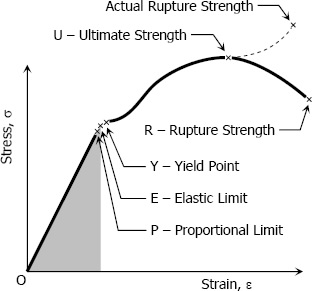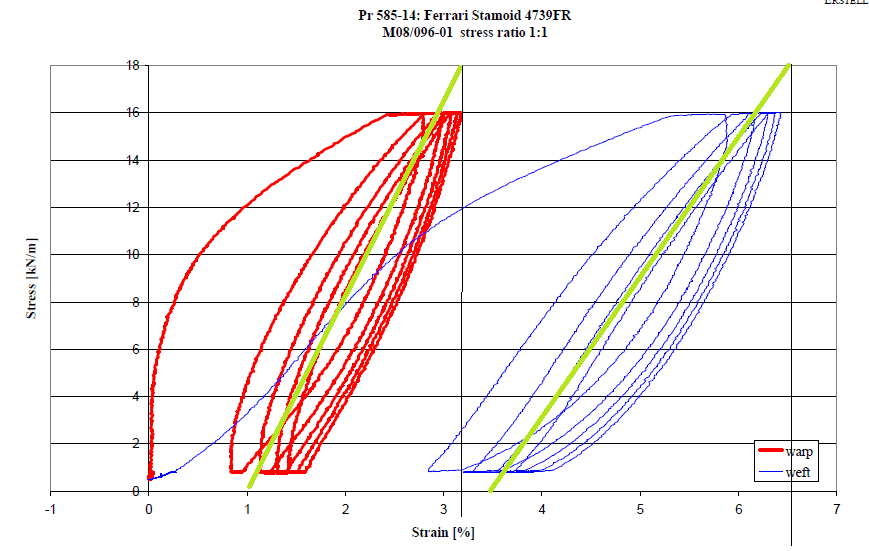In any structural calculation, we relate the deformation of the elements that make up the structure with the stresses to which they are subjected. This relationship is often expressed normally using the stress-strain graph of such material.Fig. 1: Stress-strain graph of steel

So, we know that the more you deform a structural element, more stress it acquires. This relationship stress-strain is the quantified with a value that is inherent to each material, which we call module of deformation, Young’s modulus, modulus of elasticity, etc., and it is usually represented by the letter E.

If this relation is proportional, that is to say to double the distortion corresponds to double the stress, we say that the material in question has a module of linear or constant elasticity.

If the stress-strain graph when we increase the stress coincides with the same graph when we remove the stress, we say that the material is elastic and, obviously, when we remove the stress deformation will be null.

If a material has the two properties we said above, it means it is an elastic material with a linear modulus of elasticity.

There are materials that show flexible behavior (that is to say they recover the initial form on having removed the stress) only when we do not pass a certain value of stress. If we pass this value, having removed stress on the material, the stress-strain graph will not coincide with the graph obtained on having loaded it. And we will observe that, on having removed the stress, a permanent distortion stays.

This limit stress value, beyond which the material no longer has an elastic behavior, is called elastic limit of the material. In the case of steel, this stress of the elastic limit is a value approximate to E = 210 GPa = 2,100,000 kg/cm².

##### What happens with the architectural membranes?

Each architectural membrane has its stress-strain graphic. This graph is not the same in all directions but that varies with the angle to the fibers of warp and weft. These materials are not isotropic (equal in all directions). In reality, they are orthotropic (two main directions, perpendicular to each other). Therefore in general terms, we typically have two stress-strain graphs for each material.

These graphs are neither linear nor elastic. That is to say neither the graph is a straight line, nor it coincides with the way back. In the figure below, we see two graphs corresponding to different cycles of stress increase and decrease. Red color corresponds stress applied in warp direction and the blue corresponds to stresses applied in the direction of weft.

We have the following question: what is the value of E in this architectural membrane ? Although the question is very simple the answer is very complicated.

1. In which direction, weft or warp?
2. In which cycle, the first time that we tighten the membrane, or the following ones?
3. In which tension; when it is little tightened or much tightened?
4. etc.Fig. 2: Stress-strain graph of an architectural membrane

But if we want to use this membrane in an analysis program we have to give a value of E.

In fact, there are computer programs of non-linear calculation that allow you to define the characteristic of the modulus of elasticity of a material such as given in a curve of a series of x,y coordinates. This is not the case of the WinTess3 program. At the moment this program requires a particular value of E for membrane. WinTess actually allows us to define an E for warp direction, another E for weft direction and yet another E for the direction of diagonal at 45°. If only we provide a value of E, then the three mentioned values are taken as equal.

##### Where do we find this value of E?

If we look at the majority of technical sheets for the architectural membranes, although there are multitude of values for different properties, we usually never find the value of E. Precisely because it is a value very difficult to define correctly. However, if we want to find this value for use in an analysis program, we will have to do something.

The first thing is to ask the manufacturer of the membrane a stress-strain graph of the membrane. Of course the manufacturer will tell us that there are many graphs of this type, depending on the questions that we asked in the previous paragraph.

Thus we must ask something like this:

“Stress-strain graph of a membrane in the direction of warp, when there is stress only in the warp direction, between the range of 10 and 40 kN/m”

or

“Stress-strain graph a membrane in the direction of weft, when the same tension in warp and weft directions (biaxial test) is between the range of 10 to 40 kN/m”

etc.

Once we have the graph, draw a slanted line having slope media approximating curves of membrane stress-strain. (See green lines in Figure 2 )

Then we divide the height by the base:

warp graphic, red color:
height = 18 kN/m = 1,8 T/m
base = 3,2 – 1 = 2,2 % = 0,022
height / base = 1,8 / 0.022 = 81,8 T/m

weft graphic, blue color:
height= 18 kN/m = 1,8 T/m
base = 6,5 – 3,5 = 3% = 0,03
height/ base = 1,8 / 0,03 = 60 T/m

Therefore, we will use a value of E equal to 82/60 in WinTess3.